# 3D数学 – 矩阵

• A+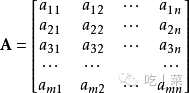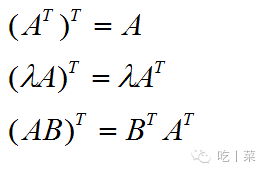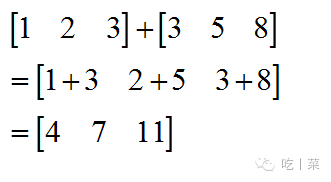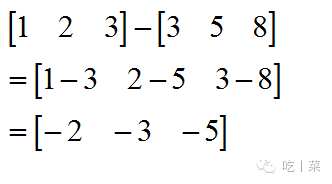A+B = B+A

(A+B)+C = A+(B+C)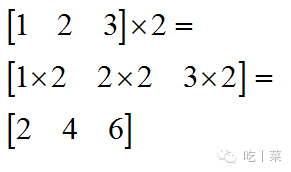( μλ )A = μ( λA )

( λ+μ )A = Aλ + μA

λ( A + B) = λA + λB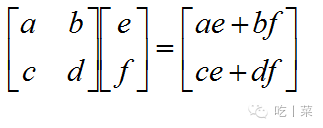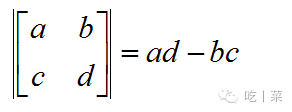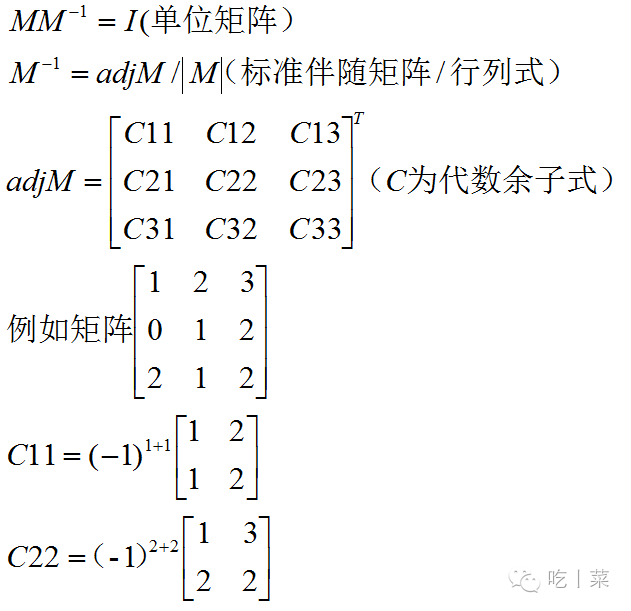• 我的微信
• 扫一扫加我微信
•• 微信我的
• 我微信扫一扫加
•